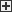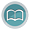Manual Chapter : What resources do I need to collect data for multiple modules

## Applies To:

Show Versions### BIG-IQ Centralized Management

• 5.4.0Use this process to estimate the number of DCDs that you need to collect and display more than one kind of data from your BIG-IP devices.

1. Perform the estimate for each module independently.
2. Record the maximum number of DCDs recommended by any one estimate.
3. Sum the total storage needed by all estimates.
4. Divide the result in step 3 by the result of step 2.

For example:

You’ve performed the estimate for an ASM module, and the result is 2 DCDs and 60 GB of storage.

You’ve performed the estimate for Statistics, and the result is 3 DCDs and 120 GB of storage.

In this example, the maximum number of DCDs is 3 and the sum of the total storage is 180 GB. Consequently, the recommendation is 3 DCDs (the maximum, from the statistics estimate) and 60 GB storage (The sum of both, divided by the recommended number of DCDs).